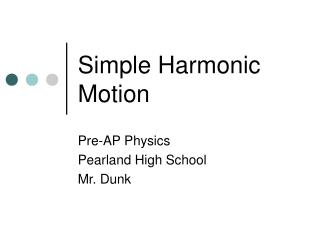DownloadDownload PresentationSimple Harmonic Motion

# Simple Harmonic Motion

Télécharger la présentation## Simple Harmonic Motion

- - - - - - - - - - - - - - - - - - - - - - - - - - - E N D - - - - - - - - - - - - - - - - - - - - - - - - - - -
##### Presentation Transcript

1. Simple Harmonic Motion Pre-AP Physics Pearland High School Mr. Dunk

2. Simple Harmonic Motion • simple harmonic motion (SHM) – vibration about an equilibrium position in which a restoring force is proportional to the displacement from equilibrium • two common types of SHM are a vibrating spring and an oscillating pendulum • springs can vibrate horizontally (on a frictionless surface) or vertically

3. Oscillating Spring

4. SHM and Oscillating Springs • in an oscillating spring, maximum velocity (with Felastic = 0) is experienced at the equilibrium point; as the spring moves away from the equilibrium point, the spring begins to exert a force that causes the velocity to decrease • the force exerted is maximum when the spring is at maximum displacement (either compressed or stretched)

5. SHM and Oscillating Springs • at maximum displacement, the velocity is zero; since the spring is either stretched or compressed at this point, a force is again exerted to start the motion over again • in an ideal system, the mass-spring system would oscillate indefinitely

6. SHM and Oscillating Springs • damping occurs when friction slows the motion of the vibrating mass, which causes the system to come to rest after a period of time • if we observe a mass-spring system over a short period of time, damping is minimal and we can assume an ideal mass-spring system

7. SHM and Oscillating Springs • in a mass-spring system, the spring force is always trying to pull or push the mass back toward equilibrium; because of this, we call this force a restoring force • in SHM, the restoring force is proportional to the mass’ displacement; this results in all SHM to be a simple back-and-forth motion over the same path

8. Hooke’s Law • in 1678, Robert Hooke proposed this simple relationship between force and displacement; Hooke’s Law is described as: Felastic = -kx • where Felastic is the spring force, • k is the spring constant • x is the maximum displacement from equilibrium

9. Hooke’s Law • the negative sign shows us that the force is a restoring force, always moving the object back to its equilibrium position • the spring constant has units of Newtons/meter • the spring constant tells us how resistant a spring is to being compressed or stretched (how many Newtons of force are required to stretch or compress the spring 1 meter) • when stretched or compressed, a spring has potential energy

10. Simple Pendulum • simple pendulum – consists of a mass (called a bob) that is attached to a fixed string; we assume that the mass of the bob is concentrated at a point at the center of mass of the bob and the mass of the string is negligible; we also disregard friction and air resistance

11. Simple Pendulum

12. Simple Pendulum • for small amplitude angles (less than 15°), a pendulum exhibits SHM • at maximum displacement from equilibrium, a pendulum bob has maximum potential energy; at equilibrium, this PE has been converted to KE • amplitude – the maximum displacement from equilibrium

13. Period and Frequency • period (T) – the time, in seconds, to execute one complete cycle of motion; units are seconds per 1 cycle • frequency (f) – the number of complete cycles of motion that occur in one second; units are cycles per 1 second (also called hertz)

14. Period and Frequency • frequency is the reciprocal of period, so • the period of a simple pendulum depends on the length of the string and the value for free-fall acceleration (in most cases, gravity)

15. Period of a Simple Pendulum • notice that only length of the string and the value for free-fall acceleration affect the period of the pendulum; period is independent of the mass of the bob or the amplitude

16. Period of a Mass-Spring System • period of a mass-spring system depends on mass and the spring constant • notice that only the mass and the spring constant affect the period of a spring; period is independent of amplitude (only for springs that obey Hooke’s Law)

17. Comparison of a Pendulum and an Oscillating Spring